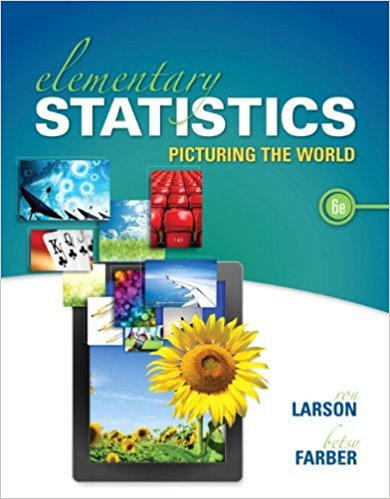×
×

# Solutions for Chapter 10.3: Comparing Two Variances## Full solutions for Elementary Statistics: Picturing the World | 6th Edition

ISBN: 9780321911216Solutions for Chapter 10.3: Comparing Two Variances

Solutions for Chapter 10.3
4 5 0 415 Reviews
26
1
##### ISBN: 9780321911216

This expansive textbook survival guide covers the following chapters and their solutions. Chapter 10.3: Comparing Two Variances includes 30 full step-by-step solutions. This textbook survival guide was created for the textbook: Elementary Statistics: Picturing the World , edition: 6. Elementary Statistics: Picturing the World was written by and is associated to the ISBN: 9780321911216. Since 30 problems in chapter 10.3: Comparing Two Variances have been answered, more than 99028 students have viewed full step-by-step solutions from this chapter.

Key Statistics Terms and definitions covered in this textbook
• 2 k factorial experiment.

A full factorial experiment with k factors and all factors tested at only two levels (settings) each.

• Analytic study

A study in which a sample from a population is used to make inference to a future population. Stability needs to be assumed. See Enumerative study

• Assignable cause

The portion of the variability in a set of observations that can be traced to speciic causes, such as operators, materials, or equipment. Also called a special cause.

• Backward elimination

A method of variable selection in regression that begins with all of the candidate regressor variables in the model and eliminates the insigniicant regressors one at a time until only signiicant regressors remain

• Biased estimator

Unbiased estimator.

• Box plot (or box and whisker plot)

A graphical display of data in which the box contains the middle 50% of the data (the interquartile range) with the median dividing it, and the whiskers extend to the smallest and largest values (or some deined lower and upper limits).

• Chi-square (or chi-squared) random variable

A continuous random variable that results from the sum of squares of independent standard normal random variables. It is a special case of a gamma random variable.

• Chi-square test

Any test of signiicance based on the chi-square distribution. The most common chi-square tests are (1) testing hypotheses about the variance or standard deviation of a normal distribution and (2) testing goodness of it of a theoretical distribution to sample data

• Conditional probability distribution

The distribution of a random variable given that the random experiment produces an outcome in an event. The given event might specify values for one or more other random variables

• Conditional probability mass function

The probability mass function of the conditional probability distribution of a discrete random variable.

• Conidence level

Another term for the conidence coeficient.

• Covariance matrix

A square matrix that contains the variances and covariances among a set of random variables, say, X1 , X X 2 k , , … . The main diagonal elements of the matrix are the variances of the random variables and the off-diagonal elements are the covariances between Xi and Xj . Also called the variance-covariance matrix. When the random variables are standardized to have unit variances, the covariance matrix becomes the correlation matrix.

• Crossed factors

Another name for factors that are arranged in a factorial experiment.

• Defect

Used in statistical quality control, a defect is a particular type of nonconformance to speciications or requirements. Sometimes defects are classiied into types, such as appearance defects and functional defects.

• Degrees of freedom.

The number of independent comparisons that can be made among the elements of a sample. The term is analogous to the number of degrees of freedom for an object in a dynamic system, which is the number of independent coordinates required to determine the motion of the object.

• Deining relation

A subset of effects in a fractional factorial design that deine the aliases in the design.

• Discrete random variable

A random variable with a inite (or countably ininite) range.

• Error sum of squares

In analysis of variance, this is the portion of total variability that is due to the random component in the data. It is usually based on replication of observations at certain treatment combinations in the experiment. It is sometimes called the residual sum of squares, although this is really a better term to use only when the sum of squares is based on the remnants of a model-itting process and not on replication.

• Finite population correction factor

A term in the formula for the variance of a hypergeometric random variable.

• Harmonic mean

The harmonic mean of a set of data values is the reciprocal of the arithmetic mean of the reciprocals of the data values; that is, h n x i n i = ? ? ? ? ? = ? ? 1 1 1 1 g .

×